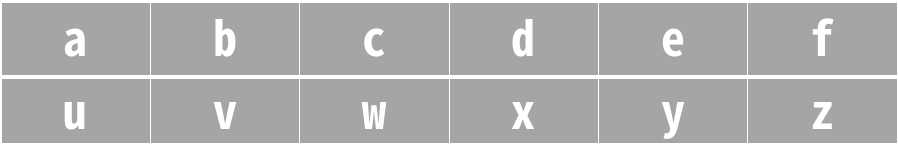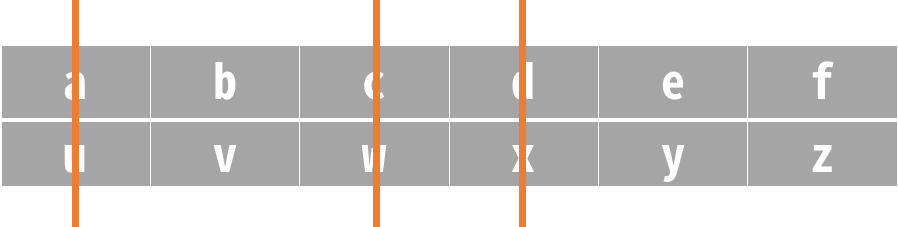# 删列造序

5 分钟读完

## 944. 删列造序 (Easy)

```输入：["cba", "daf", "ghi"]

```

```输入：["a", "b"]

```

```输入：["zyx", "wvu", "tsr"]

```

1. `1 <= A.length <= 100`
2. `1 <= A[i].length <= 1000`[贪心算法]